Next: Intersection of Lines of Up: Real-Time Symmetry Transform Previous: Real-Time Symmetry Transform

## Lines of Symmetry

The computation of lines of symmetry begins with the concept of cocircular edges. A pair of edges is cocircular if a circle can be constructed with each edge as a tangent. Furthermore, if a line is drawn connecting the centers of the edges, the angle formed between this line and each edge's tangent is the same. We define the point of cocircularity as the center of the circle (xc,yc) and its radius rc is the radius of cocircularity, as indicated in Figure. Note that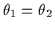in the figure.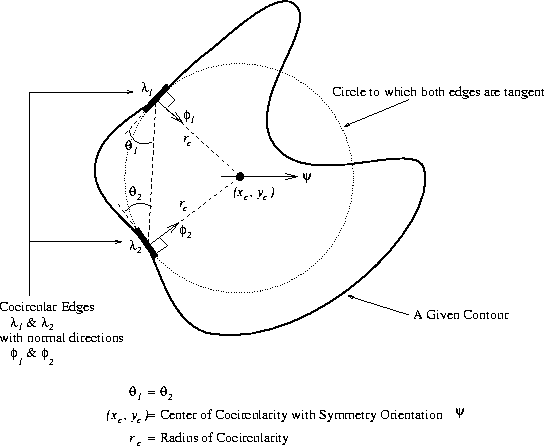Furthermore, the center of cocircularity has an orientation associated with it. This orientation is determined by the phase of the cocircular edges contributing to this center of cocircularity. The phase value of an edge is the orientation of the normal of the edge. This normal points along the direction of the change of intensity from dark to bright. As displayed in Figure, the edges have phases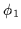and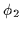which are computed from the Sobel edge detection. The symmetry orientation at the center of cocircularity,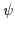, is the line that bisects the normals of the two edges. Equationreturns the value of.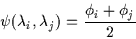(2.1)

The lines of symmetry are formed by linking all centres of circularity found in the image. In other words, circles are constructed from all pairs of cocircular edges and their centers (the centers of cocircularity) are used to trace out lines of symmetry. For each point p=(xc,yc) in the image, we consider the surrounding neighbourhood of radius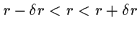. The range of r forms a ring shaped, circular annular region. Within that region, we consider all pairs of edges which have a center of cocircularity at p. We shall denote this set of cocircular edges as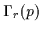. We further constrain this set of cocircular edges such that only cocircular edges with a symmetry orientation of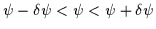are considered. Thus, this subset of edges in the neighbourhood of p is denoted as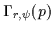.will be used to refer to the set of all pairs of cocircular edges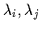with center of circularity at p with radius of cocircularityand with orientation of symmetry.

Furthermore, the centers of cocircularity can be assigned different strengths depending upon the orientation of the edges and the intensity of the edges that contribute to forming them. For each point in the image p, at each scale or radius r and for each symmetry orientationwe find the set of cocircular pairs of edges. Sela defines the magnitude of symmetry in the (p, r,) space as follows: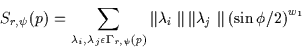(2.2)

where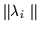and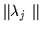are the edge intensities of the two circular edges and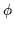is the angle separating their normals: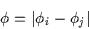(2.3)

Cocircular edges with a larger value of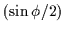, increasingly face and oppose each other and a stronger sense of symmetry is perceived at the point of cocircularity, p. The parameter w1 is used to attenuate or boost the effect of. Selecting a large w1 will diminish the contribution of non-facing edges so that only opposing cocircular edges will trigger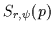. A value of w1=5 is proposed by Sela .

Thus, the magnitude of the symmetryat each point p, at each radius r and at each orientationis obtained and represents the desired lines of symmetry''. It is possible to combine the lines of symmetry from multiple radii so that an overall, r-independent value of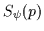is found as follows: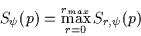(2.4)

Note that these lines of symmetry are not really lines. Rather, we compute a symmetry magnitude at each combination of p, r andso the result is a set of points with an orientation value. If true connected lines are required, these discrete points must be linked into curves using their orientation and scale value (see Chapter 3).Next: Intersection of Lines of Up: Real-Time Symmetry Transform Previous: Real-Time Symmetry Transform
Tony Jebara
2000-06-23